# `skimage.filters.rank`#

 `skimage.filters.rank.autolevel` Auto-level image using local histogram. `skimage.filters.rank.autolevel_percentile` Return grayscale local autolevel of an image. `skimage.filters.rank.enhance_contrast` Enhance contrast of an image. `skimage.filters.rank.enhance_contrast_percentile` Enhance contrast of an image. `skimage.filters.rank.entropy` Local entropy. `skimage.filters.rank.equalize` Equalize image using local histogram. `skimage.filters.rank.geometric_mean` Return local geometric mean of an image. `skimage.filters.rank.gradient` Return local gradient of an image (i.e. `skimage.filters.rank.gradient_percentile` Return local gradient of an image (i.e. `skimage.filters.rank.majority` Assign to each pixel the most common value within its neighborhood. `skimage.filters.rank.maximum` Return local maximum of an image. `skimage.filters.rank.mean` Return local mean of an image. `skimage.filters.rank.mean_bilateral` Apply a flat kernel bilateral filter. `skimage.filters.rank.mean_percentile` Return local mean of an image. `skimage.filters.rank.median` Return local median of an image. `skimage.filters.rank.minimum` Return local minimum of an image. `skimage.filters.rank.modal` Return local mode of an image. `skimage.filters.rank.noise_filter` Noise feature. `skimage.filters.rank.otsu` Local Otsu's threshold value for each pixel. `skimage.filters.rank.percentile` Return local percentile of an image. `skimage.filters.rank.pop` Return the local number (population) of pixels. `skimage.filters.rank.pop_bilateral` Return the local number (population) of pixels. `skimage.filters.rank.pop_percentile` Return the local number (population) of pixels. `skimage.filters.rank.subtract_mean` Return image subtracted from its local mean. `skimage.filters.rank.subtract_mean_percentile` Return image subtracted from its local mean. `skimage.filters.rank.sum` Return the local sum of pixels. `skimage.filters.rank.sum_bilateral` Apply a flat kernel bilateral filter. `skimage.filters.rank.sum_percentile` Return the local sum of pixels. `skimage.filters.rank.threshold` Local threshold of an image. `skimage.filters.rank.threshold_percentile` Local threshold of an image. `skimage.filters.rank.windowed_histogram` Normalized sliding window histogram

skimage.filters.rank.autolevel(image, footprint, out=None, mask=None, shift_x=False, shift_y=False, shift_z=False)[source]#

Auto-level image using local histogram.

This filter locally stretches the histogram of gray values to cover the entire range of values from “white” to “black”.

Parameters:
image([P,] M, N) ndarray (uint8, uint16)

Input image.

footprintndarray

The neighborhood expressed as an ndarray of 1’s and 0’s.

out([P,] M, N) array (same dtype as input)

If None, a new array is allocated.

Mask array that defines (>0) area of the image included in the local neighborhood. If None, the complete image is used (default).

shift_x, shift_y, shift_zint

Offset added to the footprint center point. Shift is bounded to the footprint sizes (center must be inside the given footprint).

Returns:
out([P,] M, N) ndarray (same dtype as input image)

Output image.

Examples

```>>> from skimage import data
>>> from skimage.morphology import disk, ball
>>> from skimage.filters.rank import autolevel
>>> import numpy as np
>>> img = data.camera()
>>> rng = np.random.default_rng()
>>> volume = rng.integers(0, 255, size=(10,10,10), dtype=np.uint8)
>>> auto = autolevel(img, disk(5))
>>> auto_vol = autolevel(volume, ball(5))
```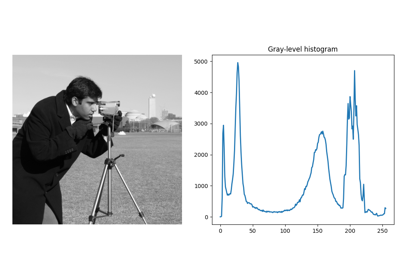Rank filters

Rank filters

skimage.filters.rank.autolevel_percentile(image, footprint, out=None, mask=None, shift_x=False, shift_y=False, p0=0, p1=1)[source]#

Return grayscale local autolevel of an image.

This filter locally stretches the histogram of grayvalues to cover the entire range of values from “white” to “black”.

Only grayvalues between percentiles [p0, p1] are considered in the filter.

Parameters:
image2-D array (uint8, uint16)

Input image.

footprint2-D array

The neighborhood expressed as a 2-D array of 1’s and 0’s.

out2-D array (same dtype as input)

If None, a new array is allocated.

Mask array that defines (>0) area of the image included in the local neighborhood. If None, the complete image is used (default).

shift_x, shift_yint

Offset added to the footprint center point. Shift is bounded to the footprint sizes (center must be inside the given footprint).

p0, p1float in [0, …, 1]

Define the [p0, p1] percentile interval to be considered for computing the value.

Returns:
out2-D array (same dtype as input image)

Output image.Rank filters

Rank filters

skimage.filters.rank.enhance_contrast(image, footprint, out=None, mask=None, shift_x=False, shift_y=False, shift_z=False)[source]#

Enhance contrast of an image.

This replaces each pixel by the local maximum if the pixel gray value is closer to the local maximum than the local minimum. Otherwise it is replaced by the local minimum.

Parameters:
image([P,] M, N) ndarray (uint8, uint16)

Input image.

footprintndarray

The neighborhood expressed as an ndarray of 1’s and 0’s.

out([P,] M, N) array (same dtype as input)

If None, a new array is allocated.

Mask array that defines (>0) area of the image included in the local neighborhood. If None, the complete image is used (default).

shift_x, shift_y, shift_zint

Offset added to the footprint center point. Shift is bounded to the footprint sizes (center must be inside the given footprint).

Returns:
out([P,] M, N) ndarray (same dtype as input image)

Output image

Examples

```>>> from skimage import data
>>> from skimage.morphology import disk, ball
>>> from skimage.filters.rank import enhance_contrast
>>> import numpy as np
>>> img = data.camera()
>>> rng = np.random.default_rng()
>>> volume = rng.integers(0, 255, size=(10,10,10), dtype=np.uint8)
>>> out = enhance_contrast(img, disk(5))
>>> out_vol = enhance_contrast(volume, ball(5))
```Rank filters

Rank filters

skimage.filters.rank.enhance_contrast_percentile(image, footprint, out=None, mask=None, shift_x=False, shift_y=False, p0=0, p1=1)[source]#

Enhance contrast of an image.

This replaces each pixel by the local maximum if the pixel grayvalue is closer to the local maximum than the local minimum. Otherwise it is replaced by the local minimum.

Only grayvalues between percentiles [p0, p1] are considered in the filter.

Parameters:
image2-D array (uint8, uint16)

Input image.

footprint2-D array

The neighborhood expressed as a 2-D array of 1’s and 0’s.

out2-D array (same dtype as input)

If None, a new array is allocated.

Mask array that defines (>0) area of the image included in the local neighborhood. If None, the complete image is used (default).

shift_x, shift_yint

Offset added to the footprint center point. Shift is bounded to the footprint sizes (center must be inside the given footprint).

p0, p1float in [0, …, 1]

Define the [p0, p1] percentile interval to be considered for computing the value.

Returns:
out2-D array (same dtype as input image)

Output image.Rank filters

Rank filters

skimage.filters.rank.entropy(image, footprint, out=None, mask=None, shift_x=False, shift_y=False, shift_z=False)[source]#

Local entropy.

The entropy is computed using base 2 logarithm i.e. the filter returns the minimum number of bits needed to encode the local gray level distribution.

Parameters:
image([P,] M, N) ndarray (uint8, uint16)

Input image.

footprintndarray

The neighborhood expressed as an ndarray of 1’s and 0’s.

out([P,] M, N) array (same dtype as input)

If None, a new array is allocated.

Mask array that defines (>0) area of the image included in the local neighborhood. If None, the complete image is used (default).

shift_x, shift_y, shift_zint

Offset added to the footprint center point. Shift is bounded to the footprint sizes (center must be inside the given footprint).

Returns:
out([P,] M, N) ndarray (float)

Output image.

References

Examples

```>>> from skimage import data
>>> from skimage.filters.rank import entropy
>>> from skimage.morphology import disk, ball
>>> import numpy as np
>>> img = data.camera()
>>> rng = np.random.default_rng()
>>> volume = rng.integers(0, 255, size=(10,10,10), dtype=np.uint8)
>>> ent = entropy(img, disk(5))
>>> ent_vol = entropy(volume, ball(5))
```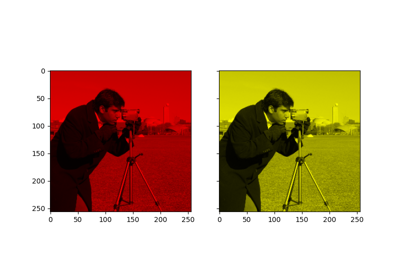Tinting gray-scale images

Tinting gray-scale images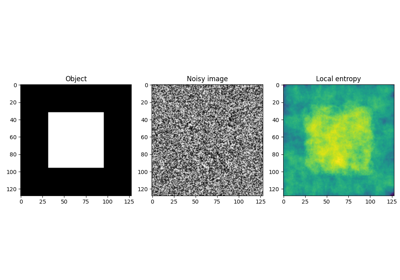Entropy

EntropyRank filters

Rank filters

skimage.filters.rank.equalize(image, footprint, out=None, mask=None, shift_x=False, shift_y=False, shift_z=False)[source]#

Equalize image using local histogram.

Parameters:
image([P,] M, N) ndarray (uint8, uint16)

Input image.

footprintndarray

The neighborhood expressed as an ndarray of 1’s and 0’s.

out([P,] M, N) array (same dtype as input)

If None, a new array is allocated.

Mask array that defines (>0) area of the image included in the local neighborhood. If None, the complete image is used (default).

shift_x, shift_y, shift_zint

Offset added to the footprint center point. Shift is bounded to the footprint sizes (center must be inside the given footprint).

Returns:
out([P,] M, N) ndarray (same dtype as input image)

Output image.

Examples

```>>> from skimage import data
>>> from skimage.morphology import disk, ball
>>> from skimage.filters.rank import equalize
>>> import numpy as np
>>> img = data.camera()
>>> rng = np.random.default_rng()
>>> volume = rng.integers(0, 255, size=(10,10,10), dtype=np.uint8)
>>> equ = equalize(img, disk(5))
>>> equ_vol = equalize(volume, ball(5))
```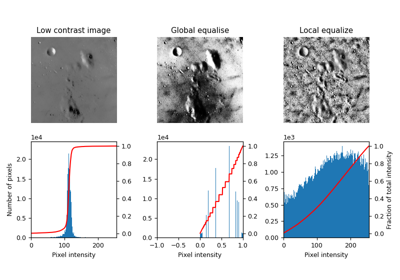Local Histogram Equalization

Local Histogram EqualizationRank filters

Rank filters

skimage.filters.rank.geometric_mean(image, footprint, out=None, mask=None, shift_x=False, shift_y=False, shift_z=False)[source]#

Return local geometric mean of an image.

Parameters:
image([P,] M, N) ndarray (uint8, uint16)

Input image.

footprintndarray

The neighborhood expressed as an ndarray of 1’s and 0’s.

out([P,] M, N) array (same dtype as input)

If None, a new array is allocated.

Mask array that defines (>0) area of the image included in the local neighborhood. If None, the complete image is used (default).

shift_x, shift_y, shift_zint

Offset added to the footprint center point. Shift is bounded to the footprint sizes (center must be inside the given footprint).

Returns:
out([P,] M, N) ndarray (same dtype as input image)

Output image.

References



Gonzalez, R. C. and Woods, R. E. “Digital Image Processing (3rd Edition).” Prentice-Hall Inc, 2006.

Examples

```>>> from skimage import data
>>> from skimage.morphology import disk, ball
>>> from skimage.filters.rank import mean
>>> import numpy as np
>>> img = data.camera()
>>> rng = np.random.default_rng()
>>> volume = rng.integers(0, 255, size=(10,10,10), dtype=np.uint8)
>>> avg = geometric_mean(img, disk(5))
>>> avg_vol = geometric_mean(volume, ball(5))
```

Return local gradient of an image (i.e. local maximum - local minimum).

Parameters:
image([P,] M, N) ndarray (uint8, uint16)

Input image.

footprintndarray

The neighborhood expressed as an ndarray of 1’s and 0’s.

out([P,] M, N) array (same dtype as input)

If None, a new array is allocated.

Mask array that defines (>0) area of the image included in the local neighborhood. If None, the complete image is used (default).

shift_x, shift_y, shift_zint

Offset added to the footprint center point. Shift is bounded to the footprint sizes (center must be inside the given footprint).

Returns:
out([P,] M, N) ndarray (same dtype as input image)

Output image.

Examples

```>>> from skimage import data
>>> from skimage.morphology import disk, ball
>>> import numpy as np
>>> img = data.camera()
>>> rng = np.random.default_rng()
>>> volume = rng.integers(0, 255, size=(10,10,10), dtype=np.uint8)
```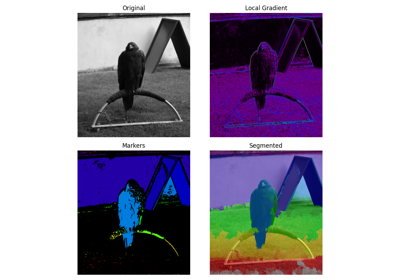Markers for watershed transform

Markers for watershed transformRank filters

Rank filters

Return local gradient of an image (i.e. local maximum - local minimum).

Only grayvalues between percentiles [p0, p1] are considered in the filter.

Parameters:
image2-D array (uint8, uint16)

Input image.

footprint2-D array

The neighborhood expressed as a 2-D array of 1’s and 0’s.

out2-D array (same dtype as input)

If None, a new array is allocated.

Mask array that defines (>0) area of the image included in the local neighborhood. If None, the complete image is used (default).

shift_x, shift_yint

Offset added to the footprint center point. Shift is bounded to the footprint sizes (center must be inside the given footprint).

p0, p1float in [0, …, 1]

Define the [p0, p1] percentile interval to be considered for computing the value.

Returns:
out2-D array (same dtype as input image)

Output image.

skimage.filters.rank.majority(image, footprint, *, out=None, mask=None, shift_x=False, shift_y=False, shift_z=False)[source]#

Assign to each pixel the most common value within its neighborhood.

Parameters:
imagendarray

Image array (uint8, uint16 array).

footprint2-D array (integer or float)

The neighborhood expressed as a 2-D array of 1’s and 0’s.

outndarray (integer or float), optional

If None, a new array will be allocated.

Mask array that defines (>0) area of the image included in the local neighborhood. If None, the complete image is used (default).

shift_x, shift_yint, optional

Offset added to the footprint center point. Shift is bounded to the footprint sizes (center must be inside the given footprint).

Returns:
out2-D array (same dtype as input image)

Output image.

Examples

```>>> from skimage import data
>>> from skimage.filters.rank import majority
>>> from skimage.morphology import disk, ball
>>> import numpy as np
>>> img = data.camera()
>>> rng = np.random.default_rng()
>>> volume = rng.integers(0, 255, size=(10,10,10), dtype=np.uint8)
>>> maj_img = majority(img, disk(5))
>>> maj_img_vol = majority(volume, ball(5))
```

skimage.filters.rank.maximum(image, footprint, out=None, mask=None, shift_x=False, shift_y=False, shift_z=False)[source]#

Return local maximum of an image.

Parameters:
image([P,] M, N) ndarray (uint8, uint16)

Input image.

footprintndarray

The neighborhood expressed as an ndarray of 1’s and 0’s.

out([P,] M, N) array (same dtype as input)

If None, a new array is allocated.

Mask array that defines (>0) area of the image included in the local neighborhood. If None, the complete image is used (default).

shift_x, shift_y, shift_zint

Offset added to the footprint center point. Shift is bounded to the footprint sizes (center must be inside the given footprint).

Returns:
out([P,] M, N) ndarray (same dtype as input image)

Output image.

Notes

The lower algorithm complexity makes `skimage.filters.rank.maximum` more efficient for larger images and footprints.

Examples

```>>> from skimage import data
>>> from skimage.morphology import disk, ball
>>> from skimage.filters.rank import maximum
>>> import numpy as np
>>> img = data.camera()
>>> rng = np.random.default_rng()
>>> volume = rng.integers(0, 255, size=(10,10,10), dtype=np.uint8)
>>> out = maximum(img, disk(5))
>>> out_vol = maximum(volume, ball(5))
```Rank filters

Rank filters

skimage.filters.rank.mean(image, footprint, out=None, mask=None, shift_x=False, shift_y=False, shift_z=False)[source]#

Return local mean of an image.

Parameters:
image([P,] M, N) ndarray (uint8, uint16)

Input image.

footprintndarray

The neighborhood expressed as an ndarray of 1’s and 0’s.

out([P,] M, N) array (same dtype as input)

If None, a new array is allocated.

Mask array that defines (>0) area of the image included in the local neighborhood. If None, the complete image is used (default).

shift_x, shift_y, shift_zint

Offset added to the footprint center point. Shift is bounded to the footprint sizes (center must be inside the given footprint).

Returns:
out([P,] M, N) ndarray (same dtype as input image)

Output image.

Examples

```>>> from skimage import data
>>> from skimage.morphology import disk, ball
>>> from skimage.filters.rank import mean
>>> import numpy as np
>>> img = data.camera()
>>> rng = np.random.default_rng()
>>> volume = rng.integers(0, 255, size=(10,10,10), dtype=np.uint8)
>>> avg = mean(img, disk(5))
>>> avg_vol = mean(volume, ball(5))
```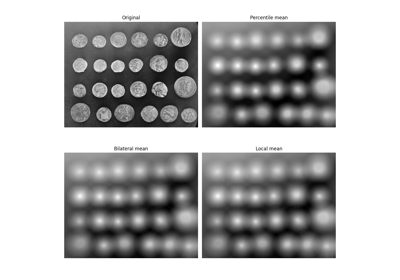Mean filters

Mean filters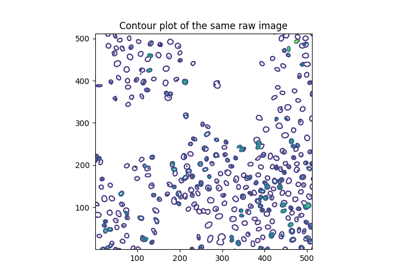Segment human cells (in mitosis)

Segment human cells (in mitosis)Rank filters

Rank filters

skimage.filters.rank.mean_bilateral(image, footprint, out=None, mask=None, shift_x=False, shift_y=False, s0=10, s1=10)[source]#

Apply a flat kernel bilateral filter.

This is an edge-preserving and noise reducing denoising filter. It averages pixels based on their spatial closeness and radiometric similarity.

Spatial closeness is measured by considering only the local pixel neighborhood given by a footprint (structuring element).

Radiometric similarity is defined by the graylevel interval [g-s0, g+s1] where g is the current pixel graylevel.

Only pixels belonging to the footprint and having a graylevel inside this interval are averaged.

Parameters:
image2-D array (uint8, uint16)

Input image.

footprint2-D array

The neighborhood expressed as a 2-D array of 1’s and 0’s.

out2-D array (same dtype as input)

If None, a new array is allocated.

Mask array that defines (>0) area of the image included in the local neighborhood. If None, the complete image is used (default).

shift_x, shift_yint

Offset added to the footprint center point. Shift is bounded to the footprint sizes (center must be inside the given footprint).

s0, s1int

Define the [s0, s1] interval around the grayvalue of the center pixel to be considered for computing the value.

Returns:
out2-D array (same dtype as input image)

Output image.

`denoise_bilateral`

Examples

```>>> import numpy as np
>>> from skimage import data
>>> from skimage.morphology import disk
>>> from skimage.filters.rank import mean_bilateral
>>> img = data.camera().astype(np.uint16)
>>> bilat_img = mean_bilateral(img, disk(20), s0=10,s1=10)
```Mean filters

Mean filtersRank filters

Rank filters

skimage.filters.rank.mean_percentile(image, footprint, out=None, mask=None, shift_x=False, shift_y=False, p0=0, p1=1)[source]#

Return local mean of an image.

Only grayvalues between percentiles [p0, p1] are considered in the filter.

Parameters:
image2-D array (uint8, uint16)

Input image.

footprint2-D array

The neighborhood expressed as a 2-D array of 1’s and 0’s.

out2-D array (same dtype as input)

If None, a new array is allocated.

Mask array that defines (>0) area of the image included in the local neighborhood. If None, the complete image is used (default).

shift_x, shift_yint

Offset added to the footprint center point. Shift is bounded to the footprint sizes (center must be inside the given footprint).

p0, p1float in [0, …, 1]

Define the [p0, p1] percentile interval to be considered for computing the value.

Returns:
out2-D array (same dtype as input image)

Output image.Mean filters

Mean filters

skimage.filters.rank.median(image, footprint=None, out=None, mask=None, shift_x=False, shift_y=False, shift_z=False)[source]#

Return local median of an image.

Parameters:
image([P,] M, N) ndarray (uint8, uint16)

Input image.

footprintndarray

The neighborhood expressed as an ndarray of 1’s and 0’s. If None, a full square of size 3 is used.

out([P,] M, N) array (same dtype as input)

If None, a new array is allocated.

Mask array that defines (>0) area of the image included in the local neighborhood. If None, the complete image is used (default).

shift_x, shift_y, shift_zint

Offset added to the footprint center point. Shift is bounded to the footprint sizes (center must be inside the given footprint).

Returns:
out([P,] M, N) ndarray (same dtype as input image)

Output image.

`skimage.filters.median`

Implementation of a median filtering which handles images with floating precision.

Examples

```>>> from skimage import data
>>> from skimage.morphology import disk, ball
>>> from skimage.filters.rank import median
>>> import numpy as np
>>> img = data.camera()
>>> rng = np.random.default_rng()
>>> volume = rng.integers(0, 255, size=(10,10,10), dtype=np.uint8)
>>> med = median(img, disk(5))
>>> med_vol = median(volume, ball(5))
```Markers for watershed transform

Markers for watershed transformRank filters

Rank filters

skimage.filters.rank.minimum(image, footprint, out=None, mask=None, shift_x=False, shift_y=False, shift_z=False)[source]#

Return local minimum of an image.

Parameters:
image([P,] M, N) ndarray (uint8, uint16)

Input image.

footprintndarray

The neighborhood expressed as an ndarray of 1’s and 0’s.

out([P,] M, N) array (same dtype as input)

If None, a new array is allocated.

Mask array that defines (>0) area of the image included in the local neighborhood. If None, the complete image is used (default).

shift_x, shift_y, shift_zint

Offset added to the footprint center point. Shift is bounded to the footprint sizes (center must be inside the given footprint).

Returns:
out([P,] M, N) ndarray (same dtype as input image)

Output image.

Notes

The lower algorithm complexity makes `skimage.filters.rank.minimum` more efficient for larger images and footprints.

Examples

```>>> from skimage import data
>>> from skimage.morphology import disk, ball
>>> from skimage.filters.rank import minimum
>>> import numpy as np
>>> img = data.camera()
>>> rng = np.random.default_rng()
>>> volume = rng.integers(0, 255, size=(10,10,10), dtype=np.uint8)
>>> out = minimum(img, disk(5))
>>> out_vol = minimum(volume, ball(5))
```Rank filters

Rank filters

skimage.filters.rank.modal(image, footprint, out=None, mask=None, shift_x=False, shift_y=False, shift_z=False)[source]#

Return local mode of an image.

The mode is the value that appears most often in the local histogram.

Parameters:
image([P,] M, N) ndarray (uint8, uint16)

Input image.

footprintndarray

The neighborhood expressed as an ndarray of 1’s and 0’s.

out([P,] M, N) array (same dtype as input)

If None, a new array is allocated.

Mask array that defines (>0) area of the image included in the local neighborhood. If None, the complete image is used (default).

shift_x, shift_y, shift_zint

Offset added to the footprint center point. Shift is bounded to the footprint sizes (center must be inside the given footprint).

Returns:
out([P,] M, N) ndarray (same dtype as input image)

Output image.

Examples

```>>> from skimage import data
>>> from skimage.morphology import disk, ball
>>> from skimage.filters.rank import modal
>>> import numpy as np
>>> img = data.camera()
>>> rng = np.random.default_rng()
>>> volume = rng.integers(0, 255, size=(10,10,10), dtype=np.uint8)
>>> out = modal(img, disk(5))
>>> out_vol = modal(volume, ball(5))
```

skimage.filters.rank.noise_filter(image, footprint, out=None, mask=None, shift_x=False, shift_y=False, shift_z=False)[source]#

Noise feature.

Parameters:
image([P,] M, N) ndarray (uint8, uint16)

Input image.

footprintndarray

The neighborhood expressed as an ndarray of 1’s and 0’s.

out([P,] M, N) array (same dtype as input)

If None, a new array is allocated.

Mask array that defines (>0) area of the image included in the local neighborhood. If None, the complete image is used (default).

shift_x, shift_y, shift_zint

Offset added to the footprint center point. Shift is bounded to the footprint sizes (center must be inside the given footprint).

Returns:
out([P,] M, N) ndarray (same dtype as input image)

Output image.

References



N. Hashimoto et al. Referenceless image quality evaluation for whole slide imaging. J Pathol Inform 2012;3:9.

Examples

```>>> from skimage import data
>>> from skimage.morphology import disk, ball
>>> from skimage.filters.rank import noise_filter
>>> import numpy as np
>>> img = data.camera()
>>> rng = np.random.default_rng()
>>> volume = rng.integers(0, 255, size=(10,10,10), dtype=np.uint8)
>>> out = noise_filter(img, disk(5))
>>> out_vol = noise_filter(volume, ball(5))
```

skimage.filters.rank.otsu(image, footprint, out=None, mask=None, shift_x=False, shift_y=False, shift_z=False)[source]#

Local Otsu’s threshold value for each pixel.

Parameters:
image([P,] M, N) ndarray (uint8, uint16)

Input image.

footprintndarray

The neighborhood expressed as an ndarray of 1’s and 0’s.

out([P,] M, N) array (same dtype as input)

If None, a new array is allocated.

Mask array that defines (>0) area of the image included in the local neighborhood. If None, the complete image is used (default).

shift_x, shift_y, shift_zint

Offset added to the footprint center point. Shift is bounded to the footprint sizes (center must be inside the given footprint).

Returns:
out([P,] M, N) ndarray (same dtype as input image)

Output image.

References

Examples

```>>> from skimage import data
>>> from skimage.filters.rank import otsu
>>> from skimage.morphology import disk, ball
>>> import numpy as np
>>> img = data.camera()
>>> rng = np.random.default_rng()
>>> volume = rng.integers(0, 255, size=(10,10,10), dtype=np.uint8)
>>> local_otsu = otsu(img, disk(5))
>>> thresh_image = img >= local_otsu
>>> local_otsu_vol = otsu(volume, ball(5))
>>> thresh_image_vol = volume >= local_otsu_vol
```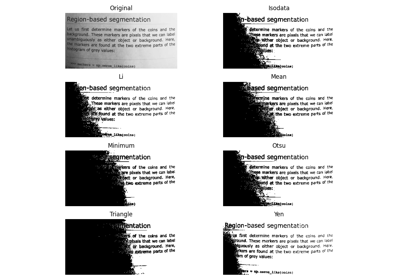Thresholding

ThresholdingRank filters

Rank filters

skimage.filters.rank.percentile(image, footprint, out=None, mask=None, shift_x=False, shift_y=False, p0=0)[source]#

Return local percentile of an image.

Returns the value of the p0 lower percentile of the local grayvalue distribution.

Only grayvalues between percentiles [p0, p1] are considered in the filter.

Parameters:
image2-D array (uint8, uint16)

Input image.

footprint2-D array

The neighborhood expressed as a 2-D array of 1’s and 0’s.

out2-D array (same dtype as input)

If None, a new array is allocated.

Mask array that defines (>0) area of the image included in the local neighborhood. If None, the complete image is used (default).

shift_x, shift_yint

Offset added to the footprint center point. Shift is bounded to the footprint sizes (center must be inside the given footprint).

p0float in [0, …, 1]

Set the percentile value.

Returns:
out2-D array (same dtype as input image)

Output image.

skimage.filters.rank.pop(image, footprint, out=None, mask=None, shift_x=False, shift_y=False, shift_z=False)[source]#

Return the local number (population) of pixels.

The number of pixels is defined as the number of pixels which are included in the footprint and the mask.

Parameters:
image([P,] M, N) ndarray (uint8, uint16)

Input image.

footprintndarray

The neighborhood expressed as an ndarray of 1’s and 0’s.

out([P,] M, N) array (same dtype as input)

If None, a new array is allocated.

Mask array that defines (>0) area of the image included in the local neighborhood. If None, the complete image is used (default).

shift_x, shift_y, shift_zint

Offset added to the footprint center point. Shift is bounded to the footprint sizes (center must be inside the given footprint).

Returns:
out([P,] M, N) ndarray (same dtype as input image)

Output image.

Examples

```>>> from skimage.morphology import square, cube # Need to add 3D example
>>> import skimage.filters.rank as rank
>>> img = 255 * np.array([[0, 0, 0, 0, 0],
...                       [0, 1, 1, 1, 0],
...                       [0, 1, 1, 1, 0],
...                       [0, 1, 1, 1, 0],
...                       [0, 0, 0, 0, 0]], dtype=np.uint8)
>>> rank.pop(img, square(3))
array([[4, 6, 6, 6, 4],
[6, 9, 9, 9, 6],
[6, 9, 9, 9, 6],
[6, 9, 9, 9, 6],
[4, 6, 6, 6, 4]], dtype=uint8)
```

skimage.filters.rank.pop_bilateral(image, footprint, out=None, mask=None, shift_x=False, shift_y=False, s0=10, s1=10)[source]#

Return the local number (population) of pixels.

The number of pixels is defined as the number of pixels which are included in the footprint and the mask. Additionally pixels must have a graylevel inside the interval [g-s0, g+s1] where g is the grayvalue of the center pixel.

Parameters:
image2-D array (uint8, uint16)

Input image.

footprint2-D array

The neighborhood expressed as a 2-D array of 1’s and 0’s.

out2-D array (same dtype as input)

If None, a new array is allocated.

Mask array that defines (>0) area of the image included in the local neighborhood. If None, the complete image is used (default).

shift_x, shift_yint

Offset added to the footprint center point. Shift is bounded to the footprint sizes (center must be inside the given footprint).

s0, s1int

Define the [s0, s1] interval around the grayvalue of the center pixel to be considered for computing the value.

Returns:
out2-D array (same dtype as input image)

Output image.

Examples

```>>> import numpy as np
>>> from skimage.morphology import square
>>> import skimage.filters.rank as rank
>>> img = 255 * np.array([[0, 0, 0, 0, 0],
...                       [0, 1, 1, 1, 0],
...                       [0, 1, 1, 1, 0],
...                       [0, 1, 1, 1, 0],
...                       [0, 0, 0, 0, 0]], dtype=np.uint16)
>>> rank.pop_bilateral(img, square(3), s0=10, s1=10)
array([[3, 4, 3, 4, 3],
[4, 4, 6, 4, 4],
[3, 6, 9, 6, 3],
[4, 4, 6, 4, 4],
[3, 4, 3, 4, 3]], dtype=uint16)
```

skimage.filters.rank.pop_percentile(image, footprint, out=None, mask=None, shift_x=False, shift_y=False, p0=0, p1=1)[source]#

Return the local number (population) of pixels.

The number of pixels is defined as the number of pixels which are included in the footprint and the mask.

Only grayvalues between percentiles [p0, p1] are considered in the filter.

Parameters:
image2-D array (uint8, uint16)

Input image.

footprint2-D array

The neighborhood expressed as a 2-D array of 1’s and 0’s.

out2-D array (same dtype as input)

If None, a new array is allocated.

Mask array that defines (>0) area of the image included in the local neighborhood. If None, the complete image is used (default).

shift_x, shift_yint

Offset added to the footprint center point. Shift is bounded to the footprint sizes (center must be inside the given footprint).

p0, p1float in [0, …, 1]

Define the [p0, p1] percentile interval to be considered for computing the value.

Returns:
out2-D array (same dtype as input image)

Output image.

skimage.filters.rank.subtract_mean(image, footprint, out=None, mask=None, shift_x=False, shift_y=False, shift_z=False)[source]#

Return image subtracted from its local mean.

Parameters:
image([P,] M, N) ndarray (uint8, uint16)

Input image.

footprintndarray

The neighborhood expressed as an ndarray of 1’s and 0’s.

out([P,] M, N) array (same dtype as input)

If None, a new array is allocated.

Mask array that defines (>0) area of the image included in the local neighborhood. If None, the complete image is used (default).

shift_x, shift_y, shift_zint

Offset added to the footprint center point. Shift is bounded to the footprint sizes (center must be inside the given footprint).

Returns:
out([P,] M, N) ndarray (same dtype as input image)

Output image.

Notes

Subtracting the mean value may introduce underflow. To compensate this potential underflow, the obtained difference is downscaled by a factor of 2 and shifted by n_bins / 2 - 1, the median value of the local histogram (n_bins = max(3, image.max()) +1 for 16-bits images and 256 otherwise).

Examples

```>>> from skimage import data
>>> from skimage.morphology import disk, ball
>>> from skimage.filters.rank import subtract_mean
>>> import numpy as np
>>> img = data.camera()
>>> rng = np.random.default_rng()
>>> volume = rng.integers(0, 255, size=(10,10,10), dtype=np.uint8)
>>> out = subtract_mean(img, disk(5))
>>> out_vol = subtract_mean(volume, ball(5))
```

skimage.filters.rank.subtract_mean_percentile(image, footprint, out=None, mask=None, shift_x=False, shift_y=False, p0=0, p1=1)[source]#

Return image subtracted from its local mean.

Only grayvalues between percentiles [p0, p1] are considered in the filter.

Parameters:
image2-D array (uint8, uint16)

Input image.

footprint2-D array

The neighborhood expressed as a 2-D array of 1’s and 0’s.

out2-D array (same dtype as input)

If None, a new array is allocated.

Mask array that defines (>0) area of the image included in the local neighborhood. If None, the complete image is used (default).

shift_x, shift_yint

Offset added to the footprint center point. Shift is bounded to the footprint sizes (center must be inside the given footprint).

p0, p1float in [0, …, 1]

Define the [p0, p1] percentile interval to be considered for computing the value.

Returns:
out2-D array (same dtype as input image)

Output image.

skimage.filters.rank.sum(image, footprint, out=None, mask=None, shift_x=False, shift_y=False, shift_z=False)[source]#

Return the local sum of pixels.

Note that the sum may overflow depending on the data type of the input array.

Parameters:
image([P,] M, N) ndarray (uint8, uint16)

Input image.

footprintndarray

The neighborhood expressed as an ndarray of 1’s and 0’s.

out([P,] M, N) array (same dtype as input)

If None, a new array is allocated.

Mask array that defines (>0) area of the image included in the local neighborhood. If None, the complete image is used (default).

shift_x, shift_y, shift_zint

Offset added to the footprint center point. Shift is bounded to the footprint sizes (center must be inside the given footprint).

Returns:
out([P,] M, N) ndarray (same dtype as input image)

Output image.

Examples

```>>> from skimage.morphology import square, cube # Need to add 3D example
>>> import skimage.filters.rank as rank         # Cube seems to fail but
>>> img = np.array([[0, 0, 0, 0, 0],            # Ball can pass
...                 [0, 1, 1, 1, 0],
...                 [0, 1, 1, 1, 0],
...                 [0, 1, 1, 1, 0],
...                 [0, 0, 0, 0, 0]], dtype=np.uint8)
>>> rank.sum(img, square(3))
array([[1, 2, 3, 2, 1],
[2, 4, 6, 4, 2],
[3, 6, 9, 6, 3],
[2, 4, 6, 4, 2],
[1, 2, 3, 2, 1]], dtype=uint8)
```

skimage.filters.rank.sum_bilateral(image, footprint, out=None, mask=None, shift_x=False, shift_y=False, s0=10, s1=10)[source]#

Apply a flat kernel bilateral filter.

This is an edge-preserving and noise reducing denoising filter. It averages pixels based on their spatial closeness and radiometric similarity.

Spatial closeness is measured by considering only the local pixel neighborhood given by a footprint (structuring element).

Radiometric similarity is defined by the graylevel interval [g-s0, g+s1] where g is the current pixel graylevel.

Only pixels belonging to the footprint AND having a graylevel inside this interval are summed.

Note that the sum may overflow depending on the data type of the input array.

Parameters:
image2-D array (uint8, uint16)

Input image.

footprint2-D array

The neighborhood expressed as a 2-D array of 1’s and 0’s.

out2-D array (same dtype as input)

If None, a new array is allocated.

Mask array that defines (>0) area of the image included in the local neighborhood. If None, the complete image is used (default).

shift_x, shift_yint

Offset added to the footprint center point. Shift is bounded to the footprint sizes (center must be inside the given footprint).

s0, s1int

Define the [s0, s1] interval around the grayvalue of the center pixel to be considered for computing the value.

Returns:
out2-D array (same dtype as input image)

Output image.

`denoise_bilateral`

Examples

```>>> import numpy as np
>>> from skimage import data
>>> from skimage.morphology import disk
>>> from skimage.filters.rank import sum_bilateral
>>> img = data.camera().astype(np.uint16)
>>> bilat_img = sum_bilateral(img, disk(10), s0=10, s1=10)
```

skimage.filters.rank.sum_percentile(image, footprint, out=None, mask=None, shift_x=False, shift_y=False, p0=0, p1=1)[source]#

Return the local sum of pixels.

Only grayvalues between percentiles [p0, p1] are considered in the filter.

Note that the sum may overflow depending on the data type of the input array.

Parameters:
image2-D array (uint8, uint16)

Input image.

footprint2-D array

The neighborhood expressed as a 2-D array of 1’s and 0’s.

out2-D array (same dtype as input)

If None, a new array is allocated.

Mask array that defines (>0) area of the image included in the local neighborhood. If None, the complete image is used (default).

shift_x, shift_yint

Offset added to the footprint center point. Shift is bounded to the footprint sizes (center must be inside the given footprint).

p0, p1float in [0, …, 1]

Define the [p0, p1] percentile interval to be considered for computing the value.

Returns:
out2-D array (same dtype as input image)

Output image.

skimage.filters.rank.threshold(image, footprint, out=None, mask=None, shift_x=False, shift_y=False, shift_z=False)[source]#

Local threshold of an image.

The resulting binary mask is True if the gray value of the center pixel is greater than the local mean.

Parameters:
image([P,] M, N) ndarray (uint8, uint16)

Input image.

footprintndarray

The neighborhood expressed as an ndarray of 1’s and 0’s.

out([P,] M, N) array (same dtype as input)

If None, a new array is allocated.

Mask array that defines (>0) area of the image included in the local neighborhood. If None, the complete image is used (default).

shift_x, shift_y, shift_zint

Offset added to the footprint center point. Shift is bounded to the footprint sizes (center must be inside the given footprint).

Returns:
out([P,] M, N) ndarray (same dtype as input image)

Output image.

Examples

```>>> from skimage.morphology import square, cube # Need to add 3D example
>>> from skimage.filters.rank import threshold
>>> img = 255 * np.array([[0, 0, 0, 0, 0],
...                       [0, 1, 1, 1, 0],
...                       [0, 1, 1, 1, 0],
...                       [0, 1, 1, 1, 0],
...                       [0, 0, 0, 0, 0]], dtype=np.uint8)
>>> threshold(img, square(3))
array([[0, 0, 0, 0, 0],
[0, 1, 1, 1, 0],
[0, 1, 0, 1, 0],
[0, 1, 1, 1, 0],
[0, 0, 0, 0, 0]], dtype=uint8)
```

skimage.filters.rank.threshold_percentile(image, footprint, out=None, mask=None, shift_x=False, shift_y=False, p0=0)[source]#

Local threshold of an image.

The resulting binary mask is True if the grayvalue of the center pixel is greater than the local mean.

Only grayvalues between percentiles [p0, p1] are considered in the filter.

Parameters:
image2-D array (uint8, uint16)

Input image.

footprint2-D array

The neighborhood expressed as a 2-D array of 1’s and 0’s.

out2-D array (same dtype as input)

If None, a new array is allocated.

Mask array that defines (>0) area of the image included in the local neighborhood. If None, the complete image is used (default).

shift_x, shift_yint

Offset added to the footprint center point. Shift is bounded to the footprint sizes (center must be inside the given footprint).

p0float in [0, …, 1]

Set the percentile value.

Returns:
out2-D array (same dtype as input image)

Output image.

skimage.filters.rank.windowed_histogram(image, footprint, out=None, mask=None, shift_x=False, shift_y=False, n_bins=None)[source]#

Normalized sliding window histogram

Parameters:
image2-D array (integer or float)

Input image.

footprint2-D array (integer or float)

The neighborhood expressed as a 2-D array of 1’s and 0’s.

out2-D array (integer or float), optional

If None, a new array is allocated.

Mask array that defines (>0) area of the image included in the local neighborhood. If None, the complete image is used (default).

shift_x, shift_yint, optional

Offset added to the footprint center point. Shift is bounded to the footprint sizes (center must be inside the given footprint).

n_binsint or None

The number of histogram bins. Will default to `image.max() + 1` if None is passed.

Returns:
out3-D array (float)

Array of dimensions (H,W,N), where (H,W) are the dimensions of the input image and N is n_bins or `image.max() + 1` if no value is provided as a parameter. Effectively, each pixel is a N-D feature vector that is the histogram. The sum of the elements in the feature vector will be 1, unless no pixels in the window were covered by both footprint and mask, in which case all elements will be 0.

Examples

```>>> from skimage import data
>>> from skimage.filters.rank import windowed_histogram
>>> from skimage.morphology import disk, ball
>>> import numpy as np
>>> img = data.camera()
>>> rng = np.random.default_rng()
>>> volume = rng.integers(0, 255, size=(10,10,10), dtype=np.uint8)
>>> hist_img = windowed_histogram(img, disk(5))
```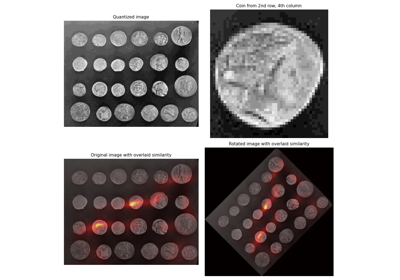Sliding window histogram

Sliding window histogram